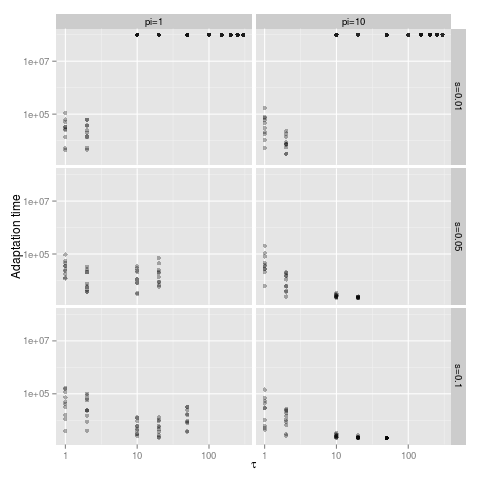# Example of using ggplot2 from IPython notebook¶

## Overview¶

The following is an example of how to use ggplot2 inside an IPython notebook.

For the data I will use the results of some evolutionary simulations I ran. As the main point here is to demonstrate the use of R and ggplot2 in the IPython noteook I will not explain what the data.

## Parse filenames¶

First I need to parse the filename for the simulation parameters.

The regular expression was written using the Python regular expression testing tool.

In :
import re
filename_pattern = pattern = re.compile('^pop_(?P<pop>\d+)_G_(?P<G>\d+)_s_(?P<s>\d\.?\d*)_H_(?P<H>\d\.?\d*)_U_(?P<U>\d\.?\d*)_beta_(?P<beta>\d\.?\d*)_pi_(?P<pi>\d\.?\d*)_tau_(?P<tau>\d\.?\d*)_(?P<date>\d{4}-\w{3}-\d{1,2})_(?P<time>\d{2}-\d{2}-\d{2}-\d{6}).(?P<extension>\w+)\$')
def parse_filename(fname):
m = pattern.match(fname)
if m:
return m.groupdict()
else:
return dict()


## Process data file¶

Next I need to read the data from file.

Data files are with .data extension and in JSON format, compressed with gzip.

You can use the builtin json parser but I use the native one I found on github by the name ultrajson because it is roughly 3-4 times faster.

In :
import ujson as json
import gzip
folder = 'output/fixation/'

def process_data_file(fname):
fpath = folder + fname
params = parse_filename(fname)
if not params:
print "Failed parsing file name", fpath
return {},[],[]
with gzip.open(fpath) as f:
if not data:
return {},[],[]
data.update(params)
W = data.pop('W')
p = data.pop('p')
data['fname'] = fname
for k in ['tau',  'G',  'H',  'pop',  'beta',  'U',  'T',  'pop_size',  's',  'pi']:
if str == type(data[k]):
data[k] = eval(data[k])
return data, W, p


Process all files into a list, each item in the list is a dict containing the results of a single simulation:

In :
import glob, os, time
tic = time.clock()
file_list = glob.glob1(folder, '*.data')
all_data = [None] * len(file_list)
print "processing", len(file_list), "data files"
for i,fname in enumerate(file_list) :
data,W,p = process_data_file(fname)
all_data[i] = data
toc = time.clock()
print "processed all files in", (toc-tic), "seconds"

processing 316 data files
processed all files in 0.34 seconds


Next I create a matrix of the values I want to plot:

In :
df = [[data['T'],data['tau'],data['s'],data['pi']] for data in all_data]


## Plotting the data with ggplot2¶

I call the rmagic extension of IPython notebook. Make sure you install rpy2, for example run: pip install rpy2.

In :
%load_ext rmagic


The final step is to send the df to R and plot the data using ggplot2. The input to R is defined by using the -i option:

In :
%%R -i df
df <- as.data.frame(df)
names(df) <- c("T","tau","s","pi")
library(ggplot2)
p <- ggplot(df, aes(tau, T))
p <- p +
geom_point(alpha=I(0.3)) +
scale_x_log10() + scale_y_log10() +
facet_grid(facets=s~pi, labeller=function(variable,value) {paste0(variable,'=',as.character(value))}) +
`